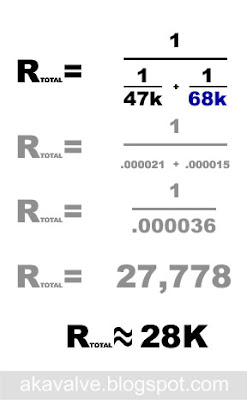## Friday, January 16, 2009

### Fender Champion 600 Fat Boost Mod Resistor Values

In the Fat Switch Mod the circuit sees 3 different resistances for the mid resistor in the tone stack: 15K (stock), 30K (Frondelli Mod fat boost value), and 47K (for a little extra boost). The actual resistors on the switch are quite different values. The 47K value is there but the other two are 68K and 22K. Why not the 15K and 30K that the circuit needs to "see" for the mod?In order to use a simpler switch I approached the mod a bit differently. I decided to replace the standard mid resistor with a 47K one. This sets the max mid resistor value. The fat switch then selects one of two resistors and connects it in parallel with the 47K resistor, lowering the effective resistance. In the center position both of the additional resistors are disconnected so the total resistance remains 47K.

This should be clear from a schematic drawing:Too find the effective resistance when one of those resistors is switched in use the formula for finding the total resistance of any number of resistors connected in parallel. Incidentally, this is the same formula you would use when connecting speakers in parallel:Since we only have two resistors connected at any time, it's a bit simpler. All we need is R1 and R2. Here's how the formula for the 47K resistor in parallel with the switched in 68K resistor is solved in detail:That 28K value is plenty close to the Frondelli Mod value of 30K. If you're wondering how close, take a look at the graph at the bottom of the Fat Switch Mod post. You'll see from comparing the curves for the three fat boost resistor values that that 2K difference doesn't matter much.

Here's the same equation for the 22K resistor in parallel with the 47K one:Solve that equation and you'll see that the 22K in parallel with the 47K results in 15K - the same effective value as the original R19. So switching in the 22K resistor puts the tone stack back to stock.Anonymous said...

But isnt Rtotal now in parallel with the original 15k resistor? So this would throw the values way off.

akavalve said...

It would be, yes. But R19 is actually removed and replaced with the resistor network. You can see it in the pix but I may not have been clear in the text. Sorry if I missed that.Anonymous said...

Got it, so on either "on" position you'll have a 30k value or a 15k value, but on the "off" position it will be 47K, which is a little more gain boost, right?

akavalve said...

Yep. You got it.